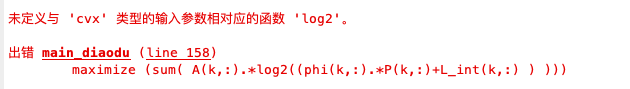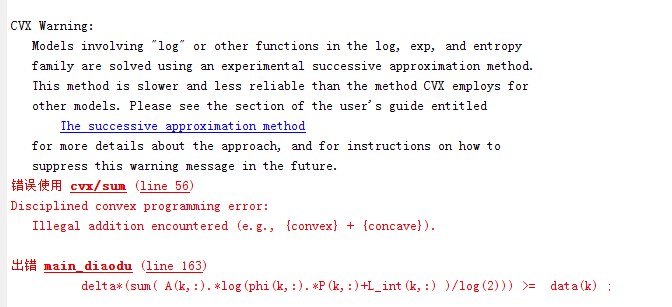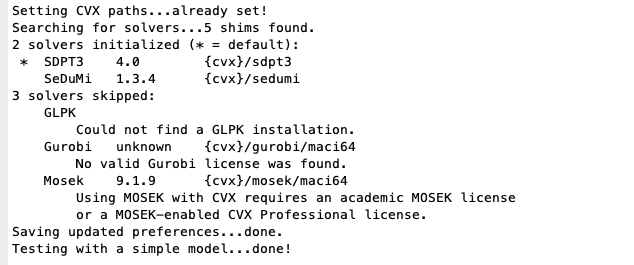# How to write log(1+Ax)? A are two constant

How to write log(1+Ax)? A are two constant.

What is a two constant?

Why not just doing

t=1+A*x;
t >= 0.0
log(t)

sorry,A is a constant
cvx can’t identify log
i want to know how to Convert log(1+Ax) to rel_entr（。 ）

You do not need to convert log(1+Ax) to rel_entr since CVX can accept log as I remember.

Post the error message you got. And maybe your complete code.

my error message is cvx can not deal with log ，i need help to convert it

I have try it,
CVX can not deal with log(1+X)
do you know how to deal with it?

You must show a full example that produces your error please. Otherwise really nobody has an idea how to help you. For example this works:

``````cvx_begin
variable X
maximize log(1+X)
subject to
X <= 10
cvx_end
``````

cvx_begin
% cvx_solver mosek
variables P(K,N)
maximize (sum( A(k,:).*log2(phi(k,:).*P(k,:)+L_int(k,) ))

``````    subject to

for k = 1:K
delta*sum(A(k,:).*(L_int(k,:)+phi(k,:).*P(k,:))) >= data2(k);
end

for k = 1:K
beta* delta*sum(  (L_int(k,:)-A(k,:))/SoD(k,:).*P(k,:) ) >= energy(k);
end

for k = 1:K
for n = 1:N
0 <= P(k,n) <=20;
end
end

for n = 1:N

P(1,n)== P(2,n) == P(3,n);
end
cvx_end
``````The function ‘log2’ corresponding to the input parameter of type ‘CVX’ is not defined.
I need help. I don’t know how to do it.

cvx_begin
% cvx_solver mosek
variables P(K,N)
maximize (sum( A(k,:).*log2(phi(k,:).*P(k,:)+L_int(k,: ) ) )

``````subject to

for k = 1:K
delta*sum(A(k,:).*(L_int(k,:)+phi(k,:).*P(k,: ) )) >= data2(k);
end
``````

for k = 1:K
beta* delta*sum( (L_int(k,:)-A(k,:))/SoD(k,:).*P(k,) >= energy(k);
end

``````for k = 1:K
for n = 1:N
0 <= P(k,n) <=20;
end
end

for n = 1:N

P(1,n)== P(2,n) == P(3,n);
end
``````

cvx_endThe function ‘log2’ corresponding to the input parameter of type ‘CVX’ is not defined.
I need help. I don’t know how to do it.

log2(x)=log(x)/log(2), according to math.

Thanks, it works.
I am a newcomer to CVX
You have been a great help to meI have encountered new problems, i need help.

I guess some elements of A(k, : ) are <= 0 but some are not. You can read the error that implied something like that. Maybe you can paste your complete code on here next time if convenient.

for K = 5

``````T = 70; N = 140; delta = T/N;
``````

w = [50,150,200,450,475;
125,100,350,300,450];

``````for c = 1:K
data(1,c) = 200;  % 初始化需要传输的数据量(bit)
end

for c = 1:K
data2(1,c) = 2^200;  % 初始化需要传输的数据量(bit)
end
for c = 1:K
energy(1,c) = 0.0001;  % 初始化需要传输的数据量(bit)
end

for c = 1:2
for n = 1:N
Q_int(c,n) = 500/N*n;  % 初始化无人机路径
end
end
``````

for k = 1:K
for n = 1:N
L_int(k,n) =1; % 初始化无人机路径
end
end

``````for a = 1:K
for n = 1:N
P_int(a,n) = 4;  % 初始化发射功率
end
end

N_0 = -100; H = 20;

% 噪声功率（单位转换）

dBm = N_0 ;
sigma = power(10, dBm/10 ) /1000 ; % 单位 w

% Unit conversion of beta_0
beta = 1000 ;

% variables
%T = 75; N = 140;
delta = T/N;

% the square of distance between UAV and kth GN in time slot n
for k = 1:K
for n = 1:N
d(k,n) =  norm( Q_int(:,n) - w(:,k) );
SoD(k,n) = power( d(k,n), 2 ) + power(H,2);
phi(k,n) = beta/sigma/SoD(k,n);              % 计算 phi_k[n]
changshu1(k,n) = log2(L_int(k,n)+phi(k,n).*P_int(k,n))
changshu2(k,n) = beta*P_int(k,n)/SoD(k,n)
end
end

% 使用CVX工具包求解调度参数
cvx_begin
``````

% cvx_solver mosek
variables A(K,N)
maximize (delta*sum( A(k,:).*changshu1(k,))
subject to

``````    % constraint 21
for k = 1:K
delta*sum(  A(k,:).*changshu1(k,:) ) >= data(k);
end

for k = 1:K
delta*sum( (L_int(k,:)-A(k,:)).*changshu2(k,:) ) >= energy(k);
end

%13 14
for n = 1:N

sum(A(:,n)) <= 1;
end

% 19 20
for k = 1:K
for n = 1:N
A(k,n) >= 0;

end
end

cvx_end
``````

E_1 = delta*sum( A(k,:).*changshu1(k,);

cvx_begin
% cvx_solver mosek
variables P(K,N)
maximize (delta*(sum( A(k,:).*(log(phi(k,:).P(k,:)+L_int(k,:)) -0.2(2^(sum(P(k,:))))))))
subject to

``````    % constraint 21
for k = 1:K
delta*(sum( A(k,:).*(log(phi(k,:).*P(k,:)+L_int(k,:))))) >=  data(k) ;

end

%13 14
for k = 1:K
beta* delta*sum(  (L_int(k,:)-A(k,:))/SoD(k,:).*P(k,:) ) >= energy(k);
end
% 19 20
for k = 1:K
for n = 1:N

0 <= P(k,n) <=20;
end
end
for n = 1:N

P(1,n)== P(2,n) == P(3,n)== P(4,n) == P(5,n);
end
cvx_end
``````

Thanks, you give me a great help.

I show my code,please check my code，thanks.

The output said it’s infeasible. Maybe it is infeasible.

It seems you use cvxquad. I doubt that is needed and it is better to use Mosek without cvxquad as described in the 2020 update on:

The output shows that CVX’s Successive Approximation method was used with SDPT3 as solver. `cvx_solver mosek` is commented out.

But as @Erling wrote, it would be better to use Mosek (with CVX 2.2) thereby avoiding CVX’s Successive Approximation method, and getting a more robust and reliable solution.

As for infeasible problems, follow the advice, except for section 1, in https://yalmip.github.io/debugginginfeasible, which also applies to CVX.

I use MAC
I have been unable to install Mosek properly
I have an official license ，but i don’t know how to use it.
Do you have any experience in this field